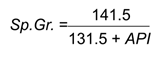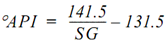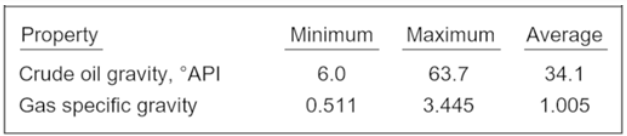# Specific Gravity & API Gravity

A specific gravity scale developed by the American Petroleum Institute (API) for measuring the relative density of various petroleum liquids, expressed in degrees. API gravity is graduated in degrees on a hydrometer instrument and was designed so that most values would fall between 10° and 70° API gravity. The arbitrary formula used to obtain this effect is presented in the following paragraphs.

Specific Gravity (SG)

Specific gravity is a dimensionless value that compares all liquids to fresh water and all gases to air.  Certain liquid physical values can be calculated by multiplying the specific gravity of that liquid by the value for fresh water. For gases, the values are calculated by multiplying the specific gravity of the gas by the value for air. Freshwater and air have a specific gravity of one. Liquids and gases with a specific gravity greater than one are heavier than water or air.

API GravityOil density is often reported in terms of API gravity. API gravity can be converted to specific gravity using the API to Specific Gravity Conversion equation, while specific gravity can be converted to API gravity using the Specific to API Gravity Conversion equation. Both equations are presented below:

API to Specific Gravity Conversion:Specific to API Gravity Conversion:### Composite Specific Gravity (SGcomp )

The composite specific gravity of oil and water mixture can be calculated if the individual fluid’s specific gravities and percentages are known. The specific gravity of this mixture is calculated as shown in the following equation:

SGcomp = [SGo × (1 – WC)] + (SGw × WC)

Where:

WC = Water Cut (fraction)

SGo = Oil specific gravity

SGw = Water specific gravity

Example:

Knowing the oil gravity, water gravity and water cut, calculate the composite SG of the produced fluids:

• Oil Gravity: 35 API,
• Water Cut: 90%,
• Water Gravity: 1,01 rel-H2O,

Using the API to Specific Gravity Conversion formula, the oil specific gravity is calculated:

SG(oil) = 141.5 / (°API+131.5) = 141.5 / (35+131.5) = 0.845.

SGo = 0.845

Then the mixture SG can be calculated:

SG= 0.9×1.01+(1-0.9)×0.845= 0.9935

SGcomp = 0.9935

NB: The following Table summarizes the ranges of data used for oil gravity and gas SG:1.chukwudi okonkwo says: